# Vlasov-Poisson-Fokker-Planck system

(diff) ← Older revision | Latest revision (diff) | Newer revision → (diff)

The linear Fokker–Planck operator is a model for a certain type of collision in a gas or plasma of particles, which are assumed to be described by a kinetic distribution function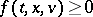. This means that the number of particles having their positions and velocities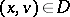at timeis given by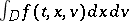. The linear Fokker–Planck collision process has been introduced by S. Chandrasekhar [a6]. The main assumption is that the collisional effects take the form of a stochastic perturbation in Newton's laws of classical mechanics, which are written as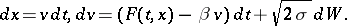Here,denotes the standard Wiener process,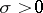is a diffusion coefficient,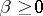is a friction parameter andis an external force (per mass unit). This random perturbation can be interpreted as the result of interactions with a thermal bath at temperature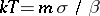, withthe mass of particles andthe Boltzmann constant. Writing the local conservation of the number of particles, one obtains the Vlasov–Fokker–Planck equation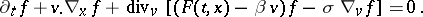The Fokker–Planck term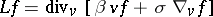can also be viewed as a simplified version of the Fokker–Planck–Landau collision operator, which is quadratic as the Boltzmann collision operator, see [a9] or [a7].

There are two situations where the Vlasov–Fokker–Planck equation is relevant: for charged particles and for gravitational systems. When dealing with a self-consistent force field, one obtains in both cases the Poisson equation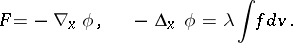The constantis positive in the Coulombic case and negative in the Newtonian case. See [a8], [a10] for discussions on this model in the latter gravitational case.

Concerning the mathematical study of the Vlasov–Poisson–Fokker–Planck system, the situation is comparable with the Vlasov–Poisson system, which is obtained when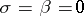. The main differences are that there are no characteristics, and that the Laplacian term gives rise to smoothing effects. Actually, the Vlasov–Fokker–Planck operator is hypo-elliptic as soon as; when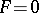, the Green function can be computed explicitly.

Weak solutions in the whole space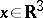,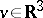can be obtained exactly as for the Vlasov–Poisson case, using an estimate of the energyExistence of strong solutions (with a bounded force) is obtained in [a2], and smoothing effects are provided in [a3]. For a bounded domain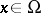,with boundary conditions, existence of weak solutions is obtained in [a5]. Concerning the asymptotics, it is proved in [a4] thattends to a stationary solution when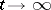(at least in the Coulombic case), by using the decrease of the free energywhich satisfiesThe same analysis has been generalized to the case of a bounded domain in [a1].

How to Cite This Entry:
Vlasov-Poisson-Fokker-Planck system. Encyclopedia of Mathematics. URL: http://encyclopediaofmath.org/index.php?title=Vlasov-Poisson-Fokker-Planck_system&oldid=16992
This article was adapted from an original article by F. Bouchut (originator), which appeared in Encyclopedia of Mathematics - ISBN 1402006098. See original article